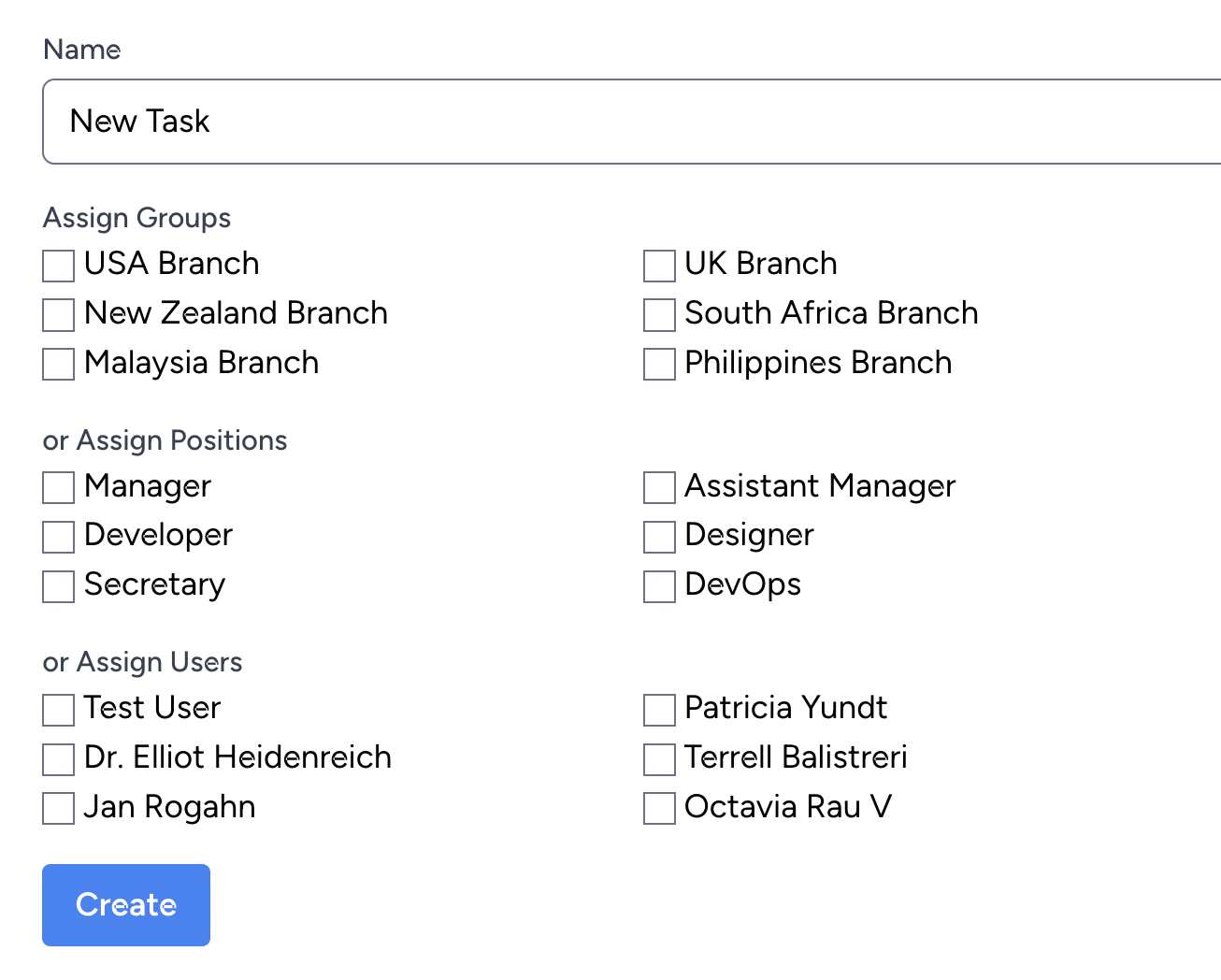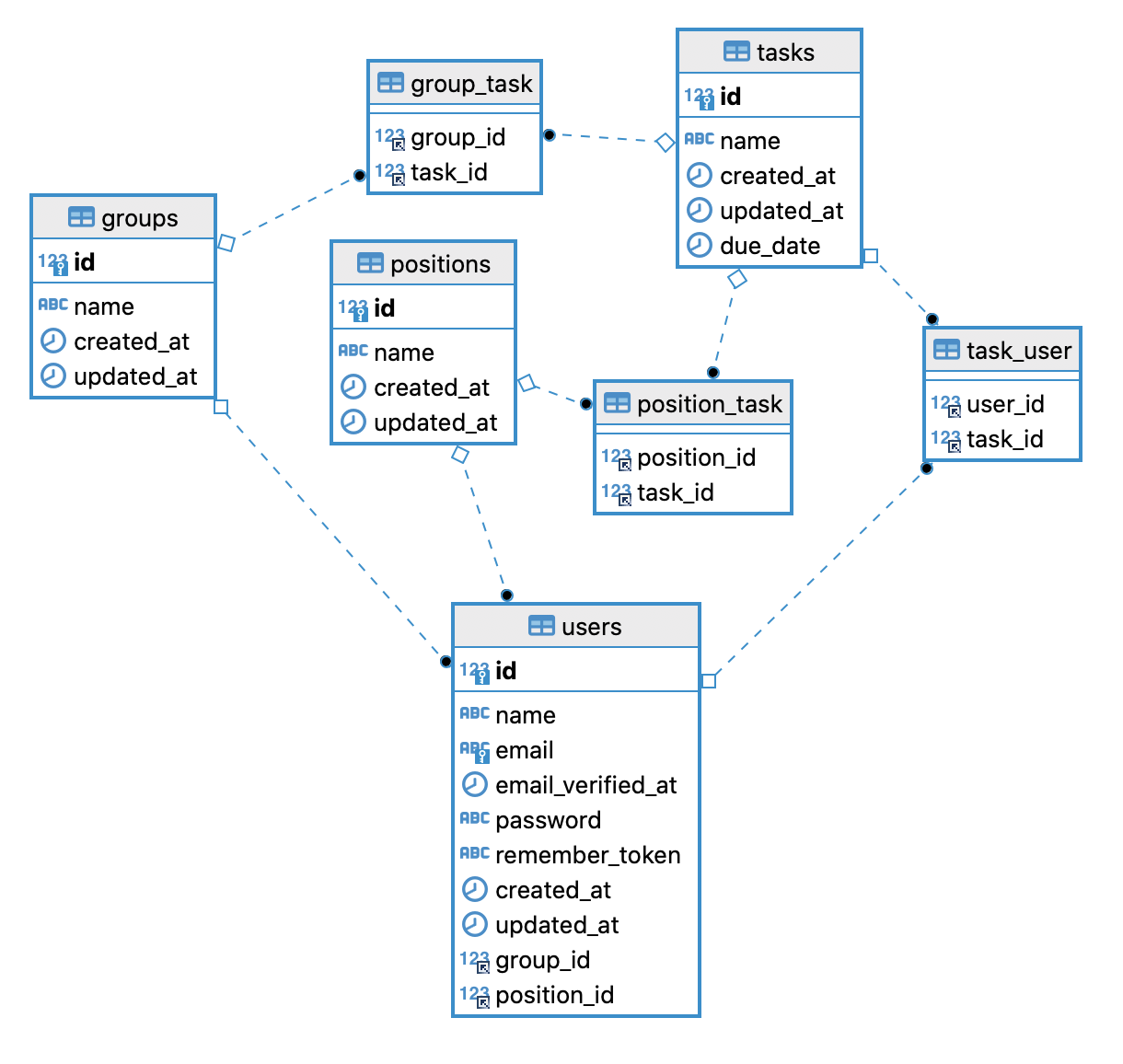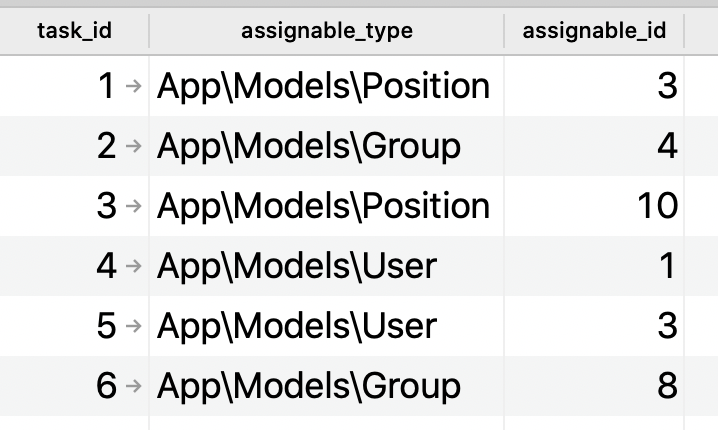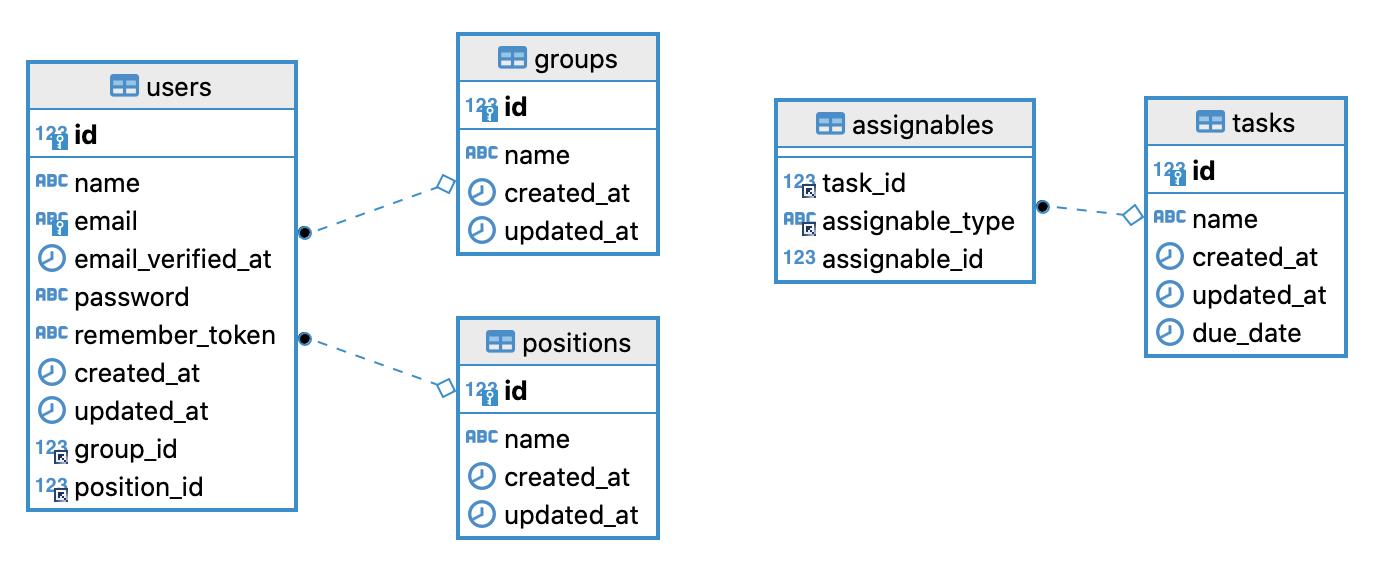# Laravel: BelongsToMany or Polymorphic Relations? Practical example.

When structuring a database, similar relationships may repeat, like a Task may be assigned to a User, User Group, or User Role. Is it worth thinking about polymorphic? In this example, let's compare the performance and convenience to use.

## Problem Explained and Two Options Shown

It's easier to understand the problem visually, so here's the form we're dealing with:So, how to save the data here? Two main options.

### Option 1. Multiple Many-to-Many Pivot Tables**

So, we can create three DB intermediate tables:

Here's the code for the Migration files:

``Schema::create('group_task', function (Blueprint \$table) {    \$table->foreignId('group_id')->constrained();    \$table->foreignId('task_id')->constrained();});Schema::create('position_task', function (Blueprint \$table) {    \$table->foreignId('position_id')->constrained();    \$table->foreignId('task_id')->constrained();});Schema::create('task_user', function (Blueprint \$table) {    \$table->foreignId('user_id')->constrained();    \$table->foreignId('task_id')->constrained();});``

And here's the Eloquent model:

``use Illuminate\Database\Eloquent\Relations\BelongsToMany; class Task extends Model{    public function users(): BelongsToMany    {        return \$this->belongsToMany(User::class);    }     public function groups(): BelongsToMany    {        return \$this->belongsToMany(Group::class);    }     public function positions(): BelongsToMany    {        return \$this->belongsToMany(Position::class);    }}``

Visually, it would look like this:### Option 2. Polymorphic in One Table

Alternatively, we may create this table with many-to-many polymorphic:

Here's the Migration code:

``Schema::create('assignables', function (Blueprint \$table) {    \$table->foreignId('task_id')->constrained();    \$table->morphs('assignable');});``

And here's the Model:

``use Illuminate\Database\Eloquent\Relations\MorphToMany; class Task extends Model{    public function users(): MorphToMany    {        return \$this->morphedByMany(User::class, 'assignable');    }     public function groups(): MorphToMany    {        return \$this->morphedByMany(Group::class, 'assignable');    }     public function positions(): MorphToMany    {        return \$this->morphedByMany(Position::class, 'assignable');    }}``

These would be the values in the DB:Visually, the DB schema would look like this:Correct, polymorphic relations are not actual DB relationships. They assign values only on the Laravel application level but not in the DB. So there are no direct foreign keys, and you can't (easily) use automatic operations like "delete on cascade".

That's actually the first drawback of polymorphic, in general. Someone could delete the Task record assigned to someone, and no related records in `assignables` would be auto-deleted or even flagged. In case of many-to-many, DB would fire the error about the constraint and prevent the Task from being deleted.

## Code for Storing Data is Identical

Now, let's look at the code for submitting the form and...

## The full tutorial [9 mins, 1766 words] is only for Premium Members

What else you will get:
• 50 courses (910 lessons, total 46 h 42 min)
• 69 long-form tutorials (one new every week)